Open in App
Not now

# How to Generate All Prime Numbers Between Two Given Numbers in Excel?

• Last Updated : 01 Dec, 2022

A number greater than 1 with no positive divisors besides 1 and the number itself is referred to as a prime number. In this article, we will be discussing two approaches for generating all prime numbers between two given numbers in excel. In the first approach, we are creating formulas for generating prime numbers between two numbers, and in the second approach, we are creating a new user-defined function that is simple and easy in comparison to the first approach.

### Approach 1: Using Formulas

The Define Name function and formulas can be used to list or generate every prime number between two certain values.

Step 1: Follow, the below steps for creating the first range name

1. Click on the Formulas tab
2. Click on Name Manager
3. Once we click on Name Manager, a new pop-up will be opened. Now, Click New Button.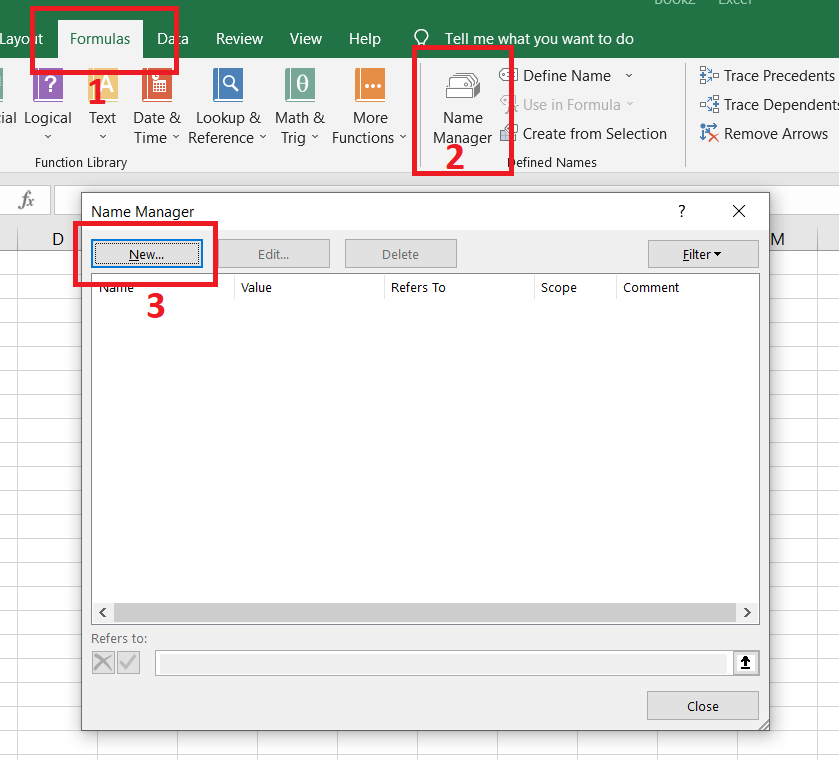Step 2: In the New Name popup window, Enter “range” as the range name in the “Name” text field. Now, Enter this formula:

=ROW(INDIRECT(Sheet1!\$B\$1&”:”&Sheet1!\$B\$2))

Sheet1 is the name of the worksheet we are now using, and B1 and B2 are the start and finish numbers we selected in the Refers to the text box.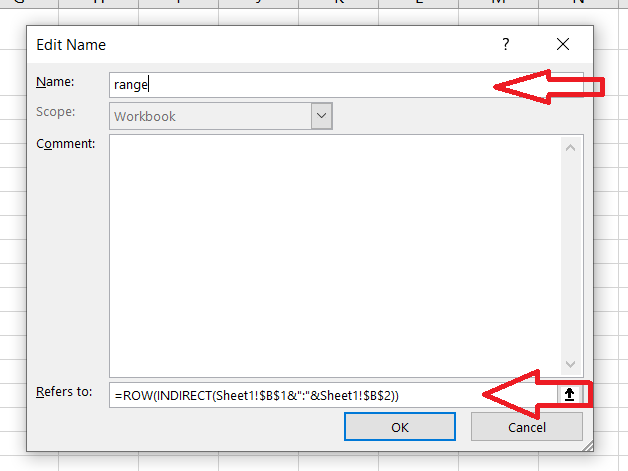Press the “OK” button and close the pop window.

Step 3: Now, Again click New Button for creating another range name. Enter “prime” as a name in Name Field in the New Name pop window. Enter this below formula in refers to the field.

=SMALL(IF(MMULT(–(IF(range>TRANSPOSE(range-Sheet1!\$B\$1+2),MOD(range,(range>TRANSPOSE(range-Sheet1!\$B\$1+2))*TRANSPOSE(range-Sheet1!\$B\$1+2)))=0), range-Sheet1!\$B\$1+2)=0,range),ROW(INDIRECT(“1:”&Sheet1!\$B\$2)))

range variable is created in step 2.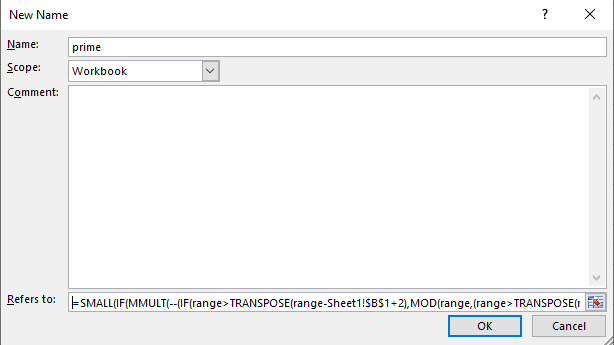Click the “OK” button and close the pop window.

Step 4: Now, Write any two numbers or ranges (B1 and B2 Column) in which range we want to print or list prime numbers as start and end numbers. We need to select any column in which we want to list or print prime numbers and we need to enter this formula in the formula bar:

=IFERROR(prime,””)

prime is a function that is created in step 3. Once we entered the formula in the formula bar and then press CTRL + SHIFT + ENTER keys together, all prime numbers will be listed in the selected column between the mentioned range.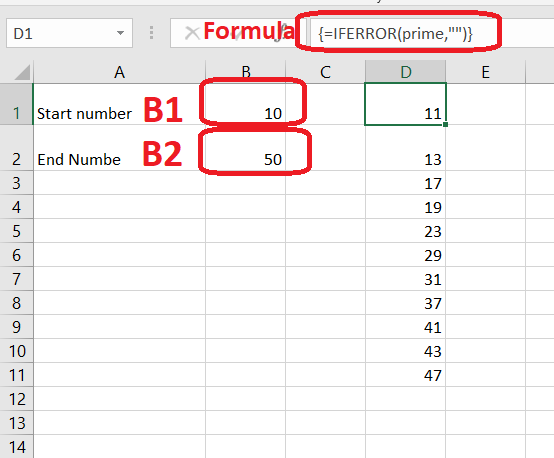### Approach 2: Using User-Defined Functions

The first method is a little bit difficult, so, in this approach, we will apply a user-defined function for generating a prime number between two numbers.

Step 1: Click the ALT + F11 keys together for opening the Microsoft Visual Basic for Applications window.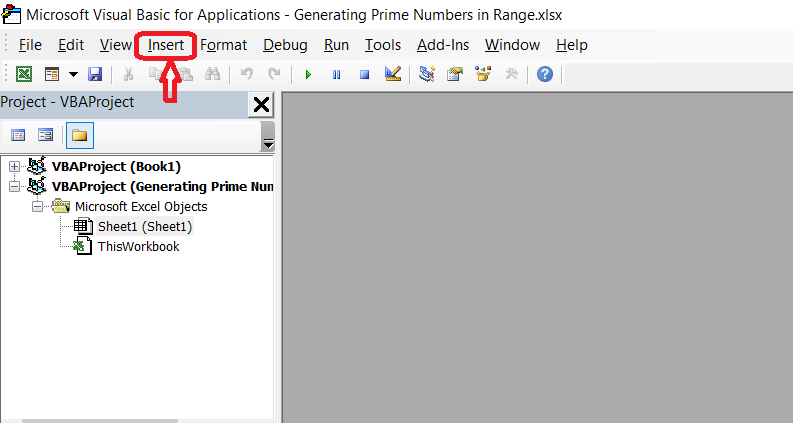Step 2: First, Click on Insert Button and then click the Module button.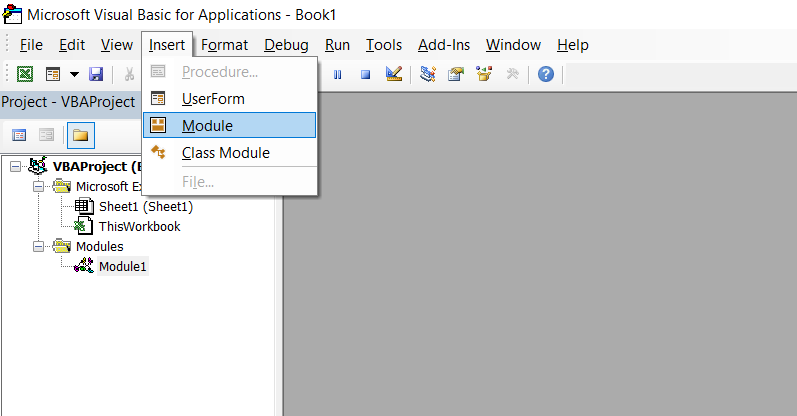Step 3: Once we clicked on the Module button, a new dialog box will open. Just copy and paste this below code to open the dialog box.

Function PRIME(Start_val, End_val As Long)

‘Updateby Extendoffice 20160613

Dim val As String

For i = Start_val To End_val

For j = 2 To i – 1

If i Mod j = 0 Then GoTo 20:

Next j

val = val & i & “,”

20:

Next i

PRIME = val

End Function

Screenshot: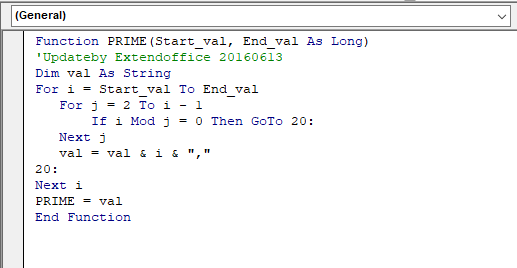Step 4: Press CTRL + S to save this file and the dialog box will be closed, go back to our worksheet. Now, Select any cell and enter this below formula with two numbers as parameters such as =prime(10, 50) where 10 is starting number and 50 is the ending number. Press ENTER Key and prime numbers will be displayed to our selected cell.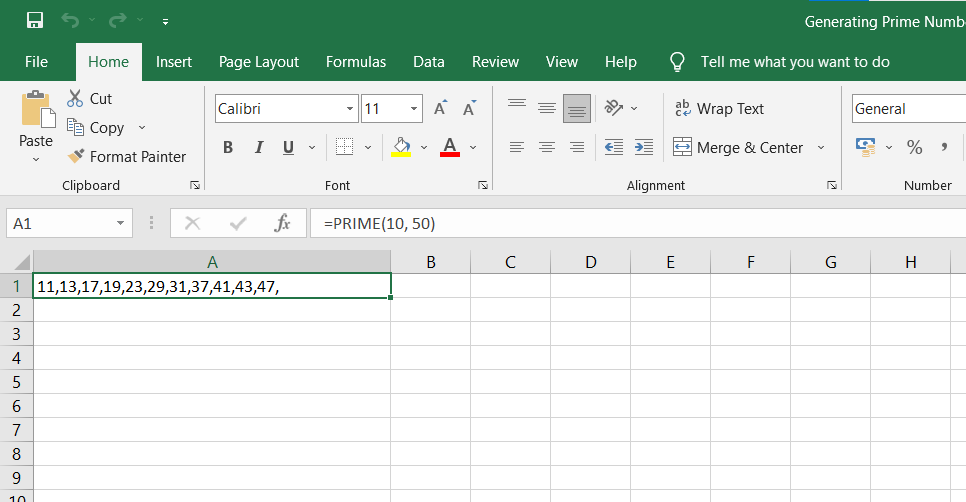My Personal Notes arrow_drop_up